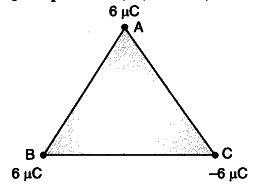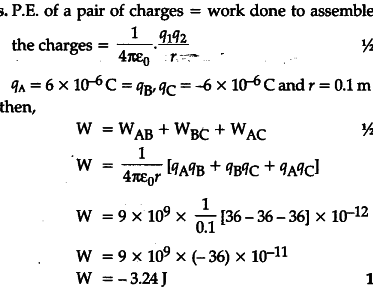# Find the amount of work done in arranging the three point charges, on the vertices of an equilateral triangle ABC, of side 10 cm, as shown in the figure

Find the amount of work done in arranging the three point charges, on the vertices of an equilateral triangle ABC, of side 10 cm, as shown in the figurehow is it 10 to the power -12 taken as common when it is supposed to be 10 to the power -18 as there is 3 terms with charges inside the bracket?